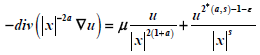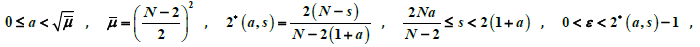# 具有Hardy项的半线性椭圆方程正径向对称解的存在性Existence of Positive Radial Symmetric Solutions for Semilinear Elliptic Equation with Hardy Exponent

DOI: 10.12677/PM.2021.113045, PDF, HTML, XML, 下载: 91  浏览: 185

Abstract: In this paper, we study the existence of positive radial symmetric solutions of, u>0 in BR(0) with Dirichlet boundary condition. Here,and 2*(a,s) is a critical exponent. We mainly prove the existence of positive radial symmetric solution of the equation by using the mountain pass lemma, Moser iteration and comparison principle.

1. 引言

$\left\{\begin{array}{l}-div\left({|x|}^{-2a}\nabla u\right)=\mu \frac{u}{{|x|}^{2\left(1+a\right)}}+\frac{{u}^{{2}^{*}\left(a,s\right)-1-\epsilon }}{{|x|}^{s}},u>0,\text{}x\in \Omega ,\\ u=0,\text{}x\in \partial \Omega ,\end{array}$ (1.1)

(1.1)是具有加权Hardy位势的半线性椭圆方程，它属于非线性微分方程。而非线性微分方程是非线性科学的主要研究方向，它在微分几何、数学物理、生态学、经济学和工程技术中都有广泛而深入的研究，而椭圆方程便是实际问题中常见的非线性微分方程，如热力学中的气体燃烧理论 、几何中的Yamabe问题 、人口动力系统 、调和分析中的Hardy-Littlewood-Sobolev不等式  等。

Cao和Peng在  中利用Moser迭代和比较原理证明了下面方程径向对称解的存在性：

$\left\{\begin{array}{l}-\Delta u=\mu \frac{u}{{|x|}^{2}}+{u}^{{2}^{*}-1-\epsilon },u>0,\text{}x\in \Omega ,\\ u=0,\text{}x\in \partial \Omega ,\end{array}$

2. 预备知识

$E\left(u\right)=\frac{1}{2}\left({\int }_{\Omega }{|x|}^{-2a}{|\nabla u|}^{2}-\mu \frac{{u}^{2}}{{|x|}^{2\left(1+a\right)}}\right)-\frac{1}{{2}^{*}\left(a,s\right)-\epsilon }{\int }_{\Omega }{|x|}^{-s}{|u|}^{{2}^{*}\left(a,s\right)-\epsilon }$$\forall u\in {H}_{0}^{1}\left(\Omega ,{|x|}^{-2a}\right)$

$‖u‖\triangleq {\left({\int }_{\Omega }{|x|}^{-2a}{|\nabla u|}^{2}-\mu \frac{{u}^{2}}{{|x|}^{2\left(1+a\right)}}\right)}^{\frac{1}{2}}$.

(1) 加权Hardy不等式

${\int }_{\Omega }\frac{{u}^{2}}{{|x|}^{2\left(1+a\right)}}\le \frac{1}{{\left(\sqrt{\stackrel{¯}{\mu }}-a\right)}^{2}}{\int }_{\Omega }\frac{{|\nabla u|}^{2}}{{|x|}^{2a}}$$\forall u\in {H}_{0}^{1}\left(\Omega ,{|x|}^{-2a}\right)$

(2) 加权Sobolev-Hardy不等式存在一个常数 $C>0$，使得

${\left({\int }_{\Omega }\frac{{u}^{q}}{{|x|}^{s}}\right)}^{1/q}\le C‖u‖$$\forall u\in {H}_{0}^{1}\left(\Omega ,{|x|}^{-2a}\right)$

(3) 对于 $q<{2}^{*}\left(a,s\right)$，从 ${H}_{0}^{1}\left(\Omega ,{|x|}^{-2a}\right)$${L}^{q}\left(\Omega \right)$ 的映射 $u\to {|x|}^{-\frac{q}{s}}u$ 是紧的。

${\left({\int }_{{ℝ}^{{}^{N}}}{|x|}^{-bq}{|u|}^{q}\right)}^{p/q}\le {C}_{a,b}{\int }_{{ℝ}^{{}^{N}}}{|x|}^{-ap}{|Du|}^{p}$

$n\le p$ 时， $-\infty $\frac{p-n}{p}$q=\frac{np}{n-p+p\left(b-a\right)}$

(1) (强制性)当 $‖u‖\to \infty$ 时， $E\left(u\right)\to \infty$，其中 $u\in M$

(2) (序列弱下半连续性)对任意 $u\in M$ 存在M中的序列 $\left\{{u}_{m}\right\}$，在V上 ${u}_{m}⇀u$ 使得

$E\left(u\right)\le \underset{m\to \infty }{\mathrm{lim}\mathrm{inf}}E\left({u}_{m}\right)$

$u\in {C}^{2}\left({B}_{R}\\left\{0\right\}\right)\cap {C}^{1}\left(\stackrel{¯}{{B}_{R}}\\left\{0\right\}\right)$ 且u满足

$\left\{\begin{array}{l}{\partial }_{i}\left({|x|}^{\beta }{\partial }_{i}u\right)+K{|x|}^{\alpha }{u}^{q}=0,\text{}\text{ }\text{ }\text{ }\text{ }\text{}x\in {B}_{R}\\left\{0\right\},\\ u>0,\text{}x\in {B}_{R}\\left\{0\right\},\\ u=0,\text{}x\in \partial {B}_{R}\\left\{0\right\},\end{array}$

3. 主要结果证明

3.1. 正解存在性证明

$\left\{\begin{array}{l}-div\left({|x|}^{-2a}\nabla u\right)=\mu \frac{u}{{|x|}^{2\left(1+a\right)}}+\frac{{u}^{{2}^{*}\left(a,s\right)-1-\epsilon }}{{|x|}^{s}},\text{}x\in \Omega ,\\ u=0,\text{}x\in \partial \Omega ,\end{array}$ (3.1)

$J\left(u\right)=\frac{1}{2}{‖u‖}^{2}-\frac{1}{{2}^{*}\left(a,s\right)-\epsilon }{\int }_{\Omega }{|x|}^{-s}{|u|}^{{2}^{*}\left(a,s\right)-\epsilon }$$u\in {H}_{0}^{1}\left(\Omega ,{|x|}^{-2a}\right)$

$|J\left({u}_{n}\right)|\le M$. (3.2)

$o\left(1\right)‖{u}_{n}‖=〈{J}^{\prime }\left({u}_{n}\right),{u}_{n}〉={‖{u}_{n}‖}^{2}-{\int }_{\Omega }{|x|}^{-s}{|{u}_{n}|}^{{2}^{*}\left(a,s\right)-\epsilon }$. (3.3)

$\begin{array}{c}M+o\left(1\right)‖{u}_{n}‖\ge \frac{1}{2}{‖{u}_{n}‖}^{2}-\frac{1}{{2}^{*}\left(a,s\right)-\epsilon }{\int }_{\Omega }{|x|}^{-s}{|u|}^{{2}^{*}\left(a,s\right)-\epsilon }\\ \text{\hspace{0.17em}}\text{\hspace{0.17em}}-\frac{1}{{2}^{*}\left(a,s\right)-\epsilon }{‖{u}_{n}‖}^{2}+\frac{1}{{2}^{*}\left(a,s\right)-\epsilon }{\int }_{\Omega }{|x|}^{-s}{|u|}^{{2}^{*}\left(a,s\right)-\epsilon }\\ =\left(\frac{1}{2}-\frac{1}{{2}^{*}\left(a,s\right)-\epsilon }\right){‖{u}_{n}‖}^{2}\end{array}$

· 在 ${H}_{0}^{1}\left(\Omega ,{|x|}^{-2a}\right)$ 中， ${u}_{n}⇀u$

· 在 ${L}^{{2}^{*}\left(a,s\right)-\epsilon }\left(\Omega \right)$ 中， ${|x|}^{-\frac{s}{{2}^{*}\left(a,s\right)-\epsilon }}{u}_{n}\to {|x|}^{-\frac{s}{{2}^{*}\left(a,s\right)-\epsilon }}u$

· ${u}_{n}$$\Omega$ 上几乎处处收敛到u。

${‖{u}_{n}{|x|}^{-\frac{s}{{2}^{*}\left(a,s\right)-\epsilon }}-u{|x|}^{-\frac{s}{{2}^{*}\left(a,s\right)-\epsilon }}‖}_{{L}^{{2}^{*}\left(a,s\right)-\epsilon }\left(\Omega \right)}\to 0$.

$〈{J}^{\prime }\left({u}_{n}\right)-{J}^{\prime }\left(u\right),{u}_{n}-u〉\le |〈{J}^{\prime }\left({u}_{n}\right),{u}_{n}-u〉|+|〈{J}^{\prime }\left(u\right),{u}_{n}-u〉|=o\left(1\right)$

$〈{J}^{\prime }\left({u}_{n}\right)-{J}^{\prime }\left(u\right),{u}_{n}-u〉={‖{u}_{n}-u‖}^{2}-{\int }_{\Omega }{|x|}^{-s}\left({|{u}_{n}|}^{{2}^{*}\left(a,s\right)-2-\epsilon }{u}_{n}-{|u|}^{{2}^{*}\left(a,s\right)-2-\epsilon }u\right)\left({u}_{n}-u\right)$.

$\begin{array}{l}{\int }_{\Omega }{|x|}^{-s}{|{u}_{n}|}^{{2}^{*}\left(a,s\right)-2-\epsilon }u\left({u}_{n}-u\right)\\ \le {\int }_{\Omega }{|x|}^{-s}{|{u}_{n}|}^{{2}^{*}\left(a,s\right)-1-\epsilon }|{u}_{n}-u|\\ ={\int }_{\Omega }{|x|}^{-s\cdot \frac{{2}^{*}\left(a,s\right)-1-\epsilon }{{2}^{*}\left(a,s\right)-\epsilon }}{|{u}_{n}|}^{{2}^{*}\left(a,s\right)-1-\epsilon }{|x|}^{-s\cdot \frac{1}{{2}^{*}\left(a,s\right)-\epsilon }}|{u}_{n}-u|\\ \le {\left({\int }_{\Omega }{|x|}^{-s}{|{u}_{n}|}^{{2}^{*}\left(a,s\right)-\epsilon }\right)}^{\frac{{2}^{*}\left(a,s\right)-1-\epsilon }{{2}^{*}\left(a,s\right)-\epsilon }}{‖{|x|}^{\frac{-s}{{2}^{*}\left(a,s\right)-\epsilon }}|{u}_{n}-u|‖}_{{L}^{{2}^{*}\left(a,s\right)-\epsilon }\left(\Omega \right)}\\ \le C{‖{u}_{n}‖}^{{2}^{*}\left(a,s\right)-1-\epsilon }{‖{u}_{n}{|x|}^{\frac{-s}{{2}^{*}\left(a,s\right)-\epsilon }}-u{|x|}^{\frac{-s}{{2}^{*}\left(a,s\right)-\epsilon }}‖}_{{L}^{{2}^{*}\left(a,s\right)-\epsilon }\left(\Omega \right)}=o\left( 1 \right)\end{array}$

$o\left(1\right)=〈{J}^{\prime }\left({u}_{n}\right)-{J}^{\prime }\left(u\right),{u}_{n}-u〉={‖{u}_{n}-u‖}^{2}+o\left(1\right)$

$c=\underset{g\in \Gamma }{\mathrm{inf}}\underset{t\in \left[0,1\right]}{\mathrm{max}}J\left(g\left(t\right)\right)$$\Gamma =\left\{g\in C\left(\left[0,1\right],{H}_{0}^{1}\left(\Omega \right)\right):g\left(0\right)=0,J\left(g\left(1\right)\right)<0\right\}$.

$J\left(u\right)=\frac{1}{2}{‖u‖}^{2}-\frac{1}{{2}^{*}\left(a,s\right)-\epsilon }{\int }_{\Omega }{|x|}^{-s}{|u|}^{{2}^{*}\left(a,s\right)-1-\epsilon }u\ge \frac{1}{2}{‖u‖}^{2}-{C}_{1}{‖u‖}^{{2}^{*}\left(a,s\right)-\epsilon }$.

$J\left(tu\right)=\frac{{t}^{2}}{2}{‖u‖}^{2}-\frac{{t}^{{2}^{*}\left(a,s\right)-\epsilon }}{{2}^{*}\left(a,s\right)-\epsilon }{\int }_{\Omega }{|x|}^{-s}{|u|}^{{2}^{*}\left(a,s\right)-1-\epsilon }u$.

3.2. 径向对称性证明

$\left\{\begin{array}{l}-div\left({|x|}^{-2a-2\nu }\nabla \upsilon \right)={|x|}^{-\left({2}^{*}\left(a,s\right)-\epsilon \right)\nu -s}{\upsilon }^{{2}^{*}\left(a,s\right)-1-\epsilon },\text{}\upsilon >0\text{}x\in \Omega ,\\ \upsilon =0\text{}x\in \partial \Omega .\end{array}$ (3.4)

$\begin{array}{c}{\int }_{\Omega }{|x|}^{-2a-2\nu }{|\nabla \upsilon |}^{2}={\int }_{\Omega }{|x|}^{-2a-2\nu }{|{|x|}^{\nu }\nabla u+\nu {|x|}^{\nu -2}ux|}^{2}\\ \le 2\left({\int }_{\Omega }{|x|}^{-2a}{|\nabla u|}^{2}+{\nu }^{2}{\int }_{\Omega }\frac{{u}^{2}}{{|x|}^{2\left(1+a\right)}}\right)\\ \le C\end{array}$

(2)根据引理2.2提到的Caffarelli-Kohn-Nirenberg不等式，得对任意 $u\left(x\right)\in {H}_{0}^{1}\left(\Omega ,{|x|}^{-2a-2\nu }\right)$

${\left({\int }_{\Omega }{|x|}^{m}{|\nabla u|}^{2}\right)}^{\frac{1}{2}}\ge {C}_{m,n}{\left({\int }_{\Omega }{|x|}^{n}{|u|}^{p\left(m,n\right)}\right)}^{\frac{1}{p\left(m,n\right)}}$, (3.5)

${\int }_{\Omega }{|x|}^{m}\nabla \upsilon \cdot \nabla \phi ={\int }_{\Omega }{|x|}^{n}{\upsilon }^{p}\phi$$\forall \phi \in {H}_{0}^{1}\left(\Omega ,{|x|}^{m}\right)$.

${\int }_{\Omega }{|x|}^{m}{|\nabla \upsilon |}^{2}{\upsilon }_{l}^{2\left(t-1\right)}+2\left(t-1\right){\int }_{\Omega }{|x|}^{m}{|\nabla {\upsilon }_{l}|}^{2}{\upsilon }_{l}^{2\left(t-1\right)}={\int }_{\Omega }{|x|}^{n}{\upsilon }^{p+1}{\upsilon }_{l}^{2\left(t-1\right)}$

$\begin{array}{c}{\left({\int }_{\Omega }{|x|}^{n}{\left(\upsilon \cdot {\upsilon }_{l}^{t-1}\right)}^{p\left(m,n\right)}\right)}^{\frac{2}{p\left(m,n\right)}}\le {C}_{m,n}^{-2}{\int }_{\Omega }{|x|}^{m}{|\nabla \left(\upsilon \cdot {\upsilon }_{l}^{t-1}\right)|}^{2}\\ \le 2{C}_{m,n}^{-2}\left({\left(t-1\right)}^{2}{\int }_{\Omega }{|x|}^{m}{|\nabla {\upsilon }_{l}|}^{2}{\upsilon }_{l}^{2\left(t-1\right)}+{\int }_{\Omega }{|x|}^{m}{|\nabla \upsilon |}^{2}{\upsilon }_{l}^{2\left(t-1\right)}\right)\\ \le 2{C}_{m,n}^{-2}t{\int }_{\Omega }{|x|}^{n}{\upsilon }^{p+2t-1}\end{array}$ (3.6)

$\left\{\begin{array}{l}p-1+2{t}_{0}=p\left(m,n\right),\\ p-1+2{t}_{j+1}=p\left(m,n\right){t}_{j},\end{array}$ (3.7)

$\left\{\begin{array}{l}{M}_{0}={\left(C\cdot {C}_{m,n}^{-2}\right)}^{\frac{p\left(m,n\right)}{2}},\\ {M}_{j+1}={\left(2{C}_{m,n}^{-2}{t}_{j}{M}_{j}\right)}^{\frac{p\left(m,n\right)}{2}},\end{array}$ (3.8)

${t}_{j}=\frac{{\left({2}^{-1}p\left(m,n\right)\right)}^{j+1}\left(p\left(m,n\right)-p-1\right)+p-1}{p\left(m,n\right)-2}$

$\begin{array}{c}{\int }_{\Omega }{|x|}^{n}{\upsilon }^{p+2{t}_{1}-1}\le {\left(2{C}_{m,n}^{-2}{t}_{0}\right)}^{\frac{p\left(m,n\right)}{2}}{\left({\int }_{\Omega }{|x|}^{n}{\upsilon }^{p+2{s}_{0}-1}\right)}^{\frac{p\left(m,n\right)}{2}}\\ \le {\left(2{C}_{m,n}^{-2}{t}_{0}\right)}^{\frac{p\left(m,n\right)}{2}}{\left({C}^{\frac{p\left(m,n\right)}{2}}{C}_{m,n}^{-p\left(m,n\right)}\right)}^{\frac{p\left(m,n\right)}{2}}\\ \le {\left(2{C}_{m,n}^{-2}{t}_{0}{M}_{0}\right)}^{\frac{p\left(m,n\right)}{2}}\\ \le {M}_{1}\end{array}$

$C\left(\Omega ,n\right)=\underset{x\in \Omega }{\mathrm{max}}{|x|}^{-n}$，并根据 $p-1+2{t}_{j+1}=p\left(m,n\right){t}_{j}$ 可得

$\begin{array}{c}{|\upsilon |}_{{L}^{p\left(m,n\right){t}_{j}}\left(\Omega \right)}\le {\left({\int }_{\Omega }{|\upsilon |}^{p\left(m,n\right){t}_{j}}{|x|}^{n}\cdot {|x|}^{-n}\right)}^{\frac{1}{p\left(m,n\right){t}_{j}}}\\ \le C{\left(\Omega ,n\right)}^{\frac{1}{p\left(m,n\right){t}_{j}}}{|\upsilon |}_{{L}^{p\left(m,n\right){t}_{j}}\left(\Omega ,{|x|}^{n}\right)}^{\frac{1}{p\left(m,n\right){t}_{j}}}\\ \le C{\left(\Omega ,n\right)}^{\frac{1}{p\left(m,n\right){t}_{j}}}{M}_{j+1}^{\frac{1}{p\left(m,n\right){t}_{j}}}\\ \le C{\left(\Omega ,n\right)}^{\frac{1}{p\left(m,n\right){t}_{j}}}{\text{e}}^{\frac{d}{p\left(m,n\right){t}_{j}}}\end{array}$

$\forall x\in {B}_{\rho }\subset \subset \Omega$ 都有 $u\left(x\right)\ge C\left(\rho \right){|x|}^{-\nu }$

$\left\{\begin{array}{l}-div\left({|x|}^{-2a}\nabla {u}_{1}\right)=\mu \frac{{u}_{1}}{{|x|}^{2\left(1+a\right)}}+f,\text{}x\in \Omega ,\\ {u}_{1}=0,\text{}x\in \partial \Omega .\end{array}$ (3.9)

$U=u-{u}_{1}$，则 $U\in {H}_{0}^{1}\left(\Omega ,{|x|}^{-2a}\right)$ 且U满足

$\left\{\begin{array}{l}-div\left({|x|}^{-2a}\nabla U\right)=\mu \frac{U}{{|x|}^{2\left(1+a\right)}}+g,\text{}x\in \Omega ,\\ U=0,\text{}x\in \partial \Omega ,\end{array}$ (3.10)

$-{\int }_{\Omega }{|x|}^{-2a}{|\nabla {U}^{-}|}^{2}=-{\int }_{\Omega }\mu \frac{{\left({U}^{-}\right)}^{2}}{{|x|}^{2\left(1+a\right)}}+{\int }_{\Omega }g{U}^{-}$.

$-div\left({|x|}^{-2a}\nabla \left({u}_{1}-C{|x|}^{-\nu }\right)\right)=\mu \frac{{u}_{1}-C{|x|}^{-\nu }}{{|x|}^{2\left(1+a\right)}}+f$.

$-{\int }_{{B}_{\rho }}{|x|}^{-2a}{|\nabla \omega |}^{2}+{\int }_{\Omega }\mu \frac{{\omega }^{2}}{{|x|}^{2\left(1+a\right)}}={\int }_{\Omega }f\omega \ge 0$.

$\omega \equiv 0$ 还有第二种证法：只需证明 $-{\int }_{{B}_{\rho }}{|x|}^{-2a}{|\nabla \omega |}^{2}+{\int }_{{B}_{\rho }}\mu \frac{{\omega }^{2}}{{|x|}^{2\left(1+a\right)}}\ge 0$ 即可。显然

$\begin{array}{c}0>-{\int }_{{B}_{\rho }}{|x|}^{-2a}{|\nabla \omega |}^{2}+{\int }_{{B}_{\rho }}\mu \frac{{\omega }^{2}}{{|x|}^{2\left(1+a\right)}}\\ ={\int }_{{B}_{\rho }}{|x|}^{-2a}\nabla \left({u}_{1}-C{|x|}^{-\nu }\right)\cdot \nabla \omega -{\int }_{{B}_{\rho }}\frac{\mu }{{|x|}^{2\left(1+a\right)}}\left({u}_{1}-C{|x|}^{-\nu }\right)\omega \\ ={\int }_{{B}_{\rho }}f\omega -C\left({\int }_{{B}_{\rho }}{|x|}^{-2a}\nabla {|x|}^{-\nu }\cdot \nabla \omega -{\int }_{{B}_{\rho }}\frac{\mu }{{|x|}^{2\left(1+a\right)}}{|x|}^{-\nu }\omega \right)\\ ={\int }_{{B}_{\rho }}f\omega +\frac{C\nu }{{\rho }^{2a+\nu +1}}{\int }_{\partial {B}_{\rho }}\omega \\ >\frac{C\nu }{{\rho }^{2a+\nu +1}}{\int }_{\partial {B}_{\rho }}\omega \ge 0\end{array}$

$\left\{\begin{array}{l}u\left(x\right){|x|}^{\nu }\ge {C}_{1},\text{}\forall x\in {\Omega }^{\prime }\in \Omega ,\\ u\left(x\right){|x|}^{\nu }\le {C}_{2},\text{}\forall x\in \Omega .\end{array}$

${\partial }_{i}\left({|x|}^{-2a-2\nu }{\partial }_{i}\upsilon \right)+{|x|}^{-\left({2}^{*}\left(a,s\right)-\epsilon \right)\nu -s}{\upsilon }^{{2}^{*}\left(a,s\right)-1-\epsilon }=0$. (3.11)

$\beta =-2a-2\nu$$\alpha =-\left({2}^{*}\left(a,s\right)-\epsilon \right)\nu -s$$q={2}^{*}\left(a,s\right)-1-\epsilon$$K=1$ 时，(3.11)显然满足引理2.4中的条件。证毕！

  Joseph, D.D. and Lundgren, T.S. (1973) Quasilinear Dirichlet Problems Driven by Positive Sources. Archive for Ra-tional Mechanics and Analysis, 49, 241-269. https://doi.org/10.1007/BF00250508  Brezis, H. and Nirenberg, L. (2010) Positive Solutions of Nonlinear Elliptic Equations Involving Critical Sobolev Exponents. Communications on Pure & Applied Mathematics, 36, 437-477. https://doi.org/10.1002/cpa.3160360405  Ekeland, I. and Ghoussoub. N. (2002) Selected New Aspects of the Calculus of Variations in the Large. Bulletin of the American Mathematical Society, 39, 207-265. https://doi.org/10.1090/S0273-0979-02-00929-1  Lieb, E.H. (1983) Sharp Constants in the Hardy-Littlewood-Sobolev and Related Inequalities. Annals of Mathematics, 118, 349-374. https://doi.org/10.2307/2007032  Cao, D. and Peng, S. (2006) Asymptotic Behavior for Elliptic Problems with Singular Coefficient and Nearly Critical Sobolev Growth. Annali di Matematicapura ed Applicata, 185, 189-205. https://doi.org/10.1007/s10231-005-0150-z  Chou, K.S. and Chu, C.W. (1993) On the Best Constant for a Weighted Sobolev-Hardy Inequality. Journal of the London Mathematical Society, 48, 137-151. https://doi.org/10.1112/jlms/s2-48.1.137  Catrina, F. and Wang, Z.Q. (2001) On the Caffarelli-Kohn-Nirenberg Inequalities: Sharp Constants, Existence (and Nonexistence), and Symmetry of Extremal Functions. Communications on Pure and Applied Mathematics, 54, 229-258. https://doi.org/10.1002/1097-0312(200102)54:2<229::AID-CPA4>3.0.CO;2-I  Struwe, M. (2008) Variational Methods. 4th Edition, Springer, Berlin Heidelberg.  Lin, C.S., Ni, W.M. and Takagi, I. (1988) Large Amplitude Stationary Solutions to a Chemotaxis System. Journal of Differential Equations, 72, 1-27. https://doi.org/10.1016/0022-0396(88)90147-7  Dupaigne, L. (2002) A Nonlinear Elliptic PDE with the In-verse-Square Potential. Journal D’analyse Mathématique, 86, 359-398. https://doi.org/10.1007/BF02786656